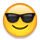Welcome! Here are the website rules, as well as some tips for using this forum.

# Horsepower per Ton Question

The horsepower per ton of a unit is .60. If the horsepower increases by 20% while the refrigeration effect decreases by 10%, what would the horsepower per ton be?

In any refrigeration system, Heat gain equals heat loss.  60% base plus 20% is 80%???  I'm not sure what you are trying to figure out? And also why your net refrigeration loss is 10%.  Is there something I'm missing?  Why are you loosing 10% and why has the HP increased by 20%.  If you plot your #'s on a pressure enthalpy, it will actually answer your Q:.

Maby Eugene can answer this Q: better.

Peace.

Mike T.
• Here you go!

Your Theoretical Horsepower per ton is equal to the mass flow rate per ton times the heat of compression (assuming no suction line superheat) divided by the constant 42.42. It will look something like this:

THp/ton = (MFR/ton x HOC) / 42.42

Since the MFR/ton is equal to 200/NRE and your COP is NRE/HOC you will get the following with a THP/ton of 0.6:

Since you said the THp/ton is 0.6, your coefficient of performance for the system will be 7.86.

A 10% decrease in the NRE will give you a 10% decrease in COP, giving you a COP of 7.074.

Backsolving this will give you a THP/ton of 0.67 with a 10% reduction in refrigeration effect.

So, in a nutshell... Let's assume  you have a  5 horsepower pump. With a THP/ton of 0.6 you will have a system capacity of 8.3 tons. If you have a 20% increase in compressor horsepower and a 10% decrease in NRE you will have a 6 hp pump operating with a THp/ton of 0.67, giving you a capacity of 9 tons...

Whew!

I'm not certain why you asked this, but I hope it helps. If you would be so kind, can you please let us know how and why this came up.... just curious!!!
• Horsepower per Ton Question
1. The question was on an exam I took. But after I had some time think about the question I was able to figure out the equation to solve for the answer.

A) 12,000 BTU/HR/TON = 20,000 BTU/HR/TON

.60 HP20,000 BTU/HR/TON = 7.85 x .10 or 10% = .785 HP/TON

2,545 BTH/HR/HP

1 TON = 12,000 BTU/HR

1 Horsepower = 2,545 BTU/HR

Sorry about that, I should've given more information as to why I asked the question. I really appreciate the assistance from my fellow HVAC mechanics.
• Correction to calculations

Your result in (A) of 20,000 should be BTU/Hr/Hp since you are dividing the 12,000 by the theoretical horsepower per ton.

So, if  you divide the 20,000 by the 2545, which is 42.42 x 60 min/hour, you will get a unitless result, which is your Coefficient of performance for the system. This is the 7.85 or 7.86 result.

In order to determine the effect of a 10% reduction in NRE, you have to multiply the 7.86 by 0.9. This will give you the COP for the 10% reduction in NRE.

The new and improved COP will be 7.074.

Now take the following:

THp/ton = [MFR/ton x HOC] / 42.42

Thp/ton = [200/NRE] x HOC / 42.42

Thp/ton = 200/(7.074 x 42.42)

Thp/ton = 0.67
• Horsepower per Ton Question

Well in this situation they were not considering COP. They just wanted the answer to be .785 or .8. I do agree the equation is not complete but this is just one of the many weird questions from a test generated by the NYC fire department. I do apprecaite the help. Thanks!
• You're Welcome....

Not a problem... Thanks for posting!
This discussion has been closed.